## 前言

C++哇，可是值类型和指针类型以及引用类型都兼顾了的！！！这里一个简单的拷贝就有很多道道！！

## =到底是 赋值还是构造

class student
{
student(const char* name, int age):name(name),age(age)
{}
student(student const& other) = default;
student& operator=(student const& other) = delete;
}
friend  std::ostream& operator <<(std::ostream& os, student& student);
private:
std::string name;
int age;
};
int main()
{
student s1("xyqin",5);
student s2=s1;             //这里到底能不能调用
s2=s1;                     //这里到底能不能调用
std::cout<<"s1:"<<s1<<std::endl;
std::cout<<"s2:"<<s2<<std::endl;
}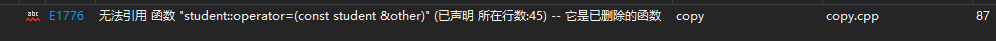student s2=s1;             //这里到底能不能调用
s2=s1;                     //这里到底能不能调用


1. student s2=s1; 这里 s2还没有构造成功，还在娘胎里，这里不是（拷贝）赋值而是在调用（拷贝）构造函数
2. s2=s1; 这里当然实在调用赋值的=,但是默认的被我们删除了，这里就提示错误了

    student(student& other)
{
std::cout << "调用拷贝构造函数" << std::endl;
this->name = other.name;
this->age = other.age;
}
student& operator=(student const& other)
{
std::cout << "调用赋值拷贝" << std::endl;
this->name = other.name;
this->age = 999;  //加一点料，标记一下
retrun *this;
}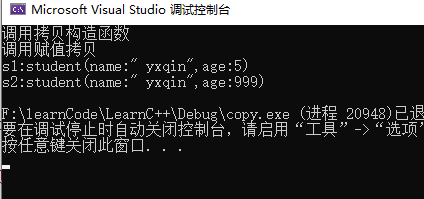## explicit 阻止隐式的转换

student(char const* info)
{
std::cout << "使用字符串构造" << std::endl;
auto tmp = std::string(info);
int index = tmp.find(';');

if (index != std::string::npos)
{
this->name = tmp.substr(0, index);
try
{
this->age = stoi(tmp.substr(index + 1, tmp.length() - index));
}
catch(...)
{
this->age = 0;
}
}
else
{
this->name = "someone";
this->age = 0;
}
}
int main()
{
student s3 = "yxqin;15";
return  0;
}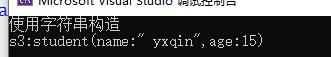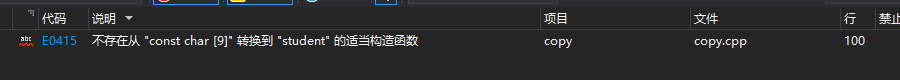student s3 = student("yxqin;15");


## 传值VS传引用

student& operator=(student const& other)


perator=(student other)


    student s4 = student("name",15 );
s4 = "yxqin;15";//没有 加  explicit


    student s1("yxqin", 5);
test_student(s1);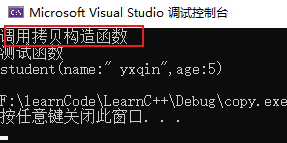student& operator=(student const& other)  //const 表示不能修改
{
//代码
retrun *this;//返回 &*this
}


## 深拷贝这么麻烦，我也不能说C+ +垃圾

class buffer
{
public:
//explicit 声明 构造器位显示调用！ 阻止隐式转换， 万一不小心把int 类型转换成了 buf类型
explicit buffer(int capacity) :capacity(capacity), len(0), buf(new unsigned char[capacity] {0})
{}
~buffer()
{
delete[] buf;
}
bool push(unsigned char value)
{
if (len == capacity) return false;
buf[len++] = value;
return  true;
}

buffer(buffer const& other) = default;
buffer& operator=(buffer const& other) = default;
friend  std::ostream& operator <<(std::ostream& os, buffer& buffer);

private:
int capacity;
int len;
unsigned char* buf;
};

std::ostream& operator <<(std::ostream& os, buffer& buffer)
{
os << "buffer(" << buffer.len << ',' << buffer.capacity << ")[";
if(buffer.buf)
for (int i = 0; i < buffer.capacity; i++)
{
os << (int)buffer.buf[i] << ',';
}
os << ']';
return os;
}


    buffer b1(15);
buffer b2 = b1;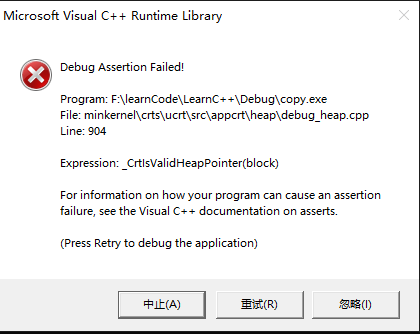buffer b1(15);
buffer b2 = b1;
b1.push(1);
std::cout<<"b1:"<<b1<< std::endl;
std::cout << "b2:" << b2 << std::endl;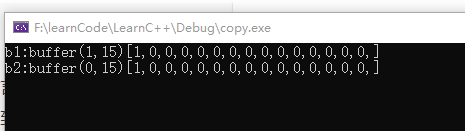buffer(buffer const& other) = default;
buffer& operator=(buffer const& other) = default;


    buffer(buffer const& other)
{
this->capacity = other.capacity;
this->len = other.len;
this->buf = new unsigned char[capacity] {};
std::copy(other.buf, other.buf + other.capacity, this->buf);
}
buffer& operator=(buffer const& other)
{
if (this == &other)return *this;
this->capacity = other.capacity;
this->len = other.len;
delete[] this->buf;// 注意删除原来的数据
this->buf = new unsigned char[capacity] {};
std::copy(other.buf, other.buf + other.capacity, this->buf);
return *this;
}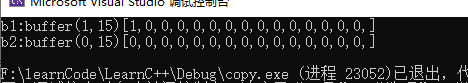## 移动构造器

    explicit buffer(int capacity) :capacity(capacity), len(0), buf(new unsigned char[capacity] {0})
{
std::cout << "调用构造函数" <<*this <<std::endl;
}
~buffer()
{
std::cout << "调用析构函数" << *this << std::endl;
delete[] buf;
}
buffer(buffer const& other)
{
std::cout << "调用拷贝构造函数" << other << std::endl;
this->capacity = other.capacity;
this->len = other.len;
this->buf = new unsigned char[capacity] {};
std::copy(other.buf, other.buf + other.capacity, this->buf);
}
buffer& operator=(buffer const& other)
{
std::cout << "调用拷贝赋值函数" << other << std::endl;
if (this == &other)return *this;
this->capacity = other.capacity;
this->len = other.len;
delete[] this->buf;// 注意删除原来的数据
this->buf = new unsigned char[capacity] {};
std::copy(other.buf, other.buf + other.capacity, this->buf);
return *this;
}
friend  std::ostream& operator <<(std::ostream& os,buffer const& buffer); //传入静态引用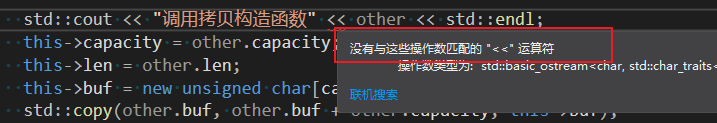buffer b1(15);
buffer b2(0);
b2 = buffer(11);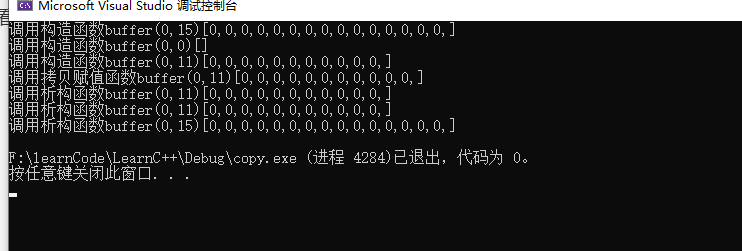b2 = buffer(11); 我们仅仅是想把buffer(11)这个临时对象覆盖掉原有的b2 ,这样 buffer11的对象应该只有一个，看看运行结果c++可不是这么想的，大致流程如下：

1. 构造临时对象buffer(11)
2. 调用拷贝构造函数传给 operator=
3. 然后调用operator= 赋值给 b2.
4. 最后临时构造的对象活不过这条代码就又要被析构了
5. 最后程序结束，析构b2

    buffer& operator=(buffer && other) noexcept
{
std::cout << "调用移动赋值函数" << other << std::endl;
if (this == &other)return *this;
//直接将 other 变成我的
this->capacity = other.capacity;
this->len = other.len;
this->buf = other.buf;

//other 会被析构掉 别忘了 该 other.buf的指向
other.buf = nullptr;
return *this;
}
buffer(buffer && other)noexcept
{
std::cout << "调用移动构造器" << other << std::endl;
//直接将 other 变成我的
this->capacity = other.capacity;
this->len = other.len;
this->buf = other.buf;

//other 会被析构掉 别忘了 该 other.buf的指向
other.buf = nullptr;
}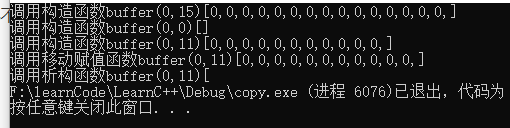### 非常有必要

    auto buffers = std::vector<buffer>();
buffers.push_back(buffer(10));
buffers.push_back(buffer(9));
buffers.push_back(buffer(8));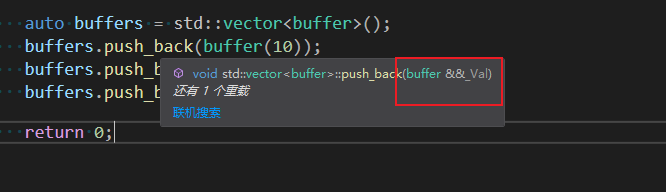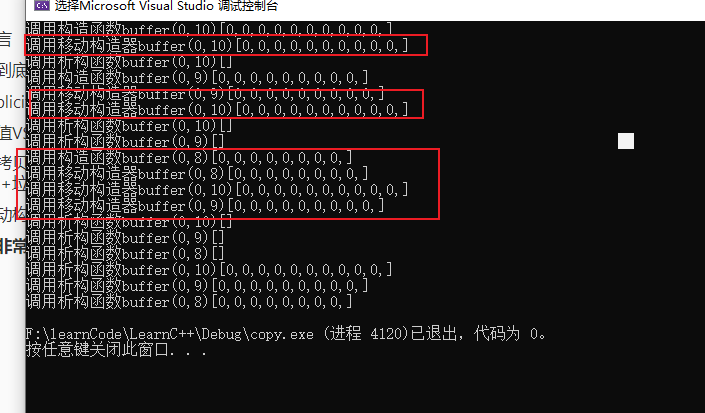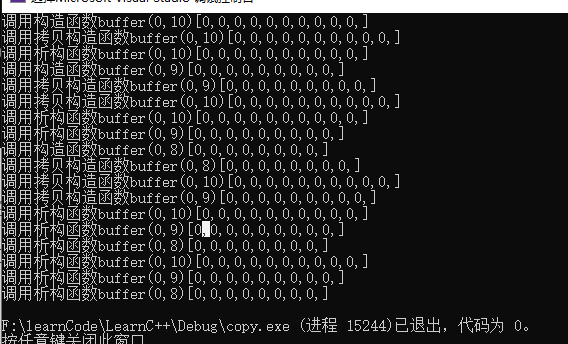## 经典的swap & copy 模式

    static void Swap(buffer& lhs, buffer& rhs)noexcept
{
std::swap(lhs.buf, rhs.buf);
std::swap(lhs.capacity, rhs.capacity);
std::swap(lhs.len, rhs.len);
}
buffer(buffer& buffer)
:capacity(buffer.capacity), len(buffer.len),
buf(capacity ? new unsigned char[capacity] {0} : nullptr)
{
if(capacity)
std::copy(buffer.buf, buffer.buf + buffer.capacity, this->buf);
std::cout << "调用拷贝构造函数" << *this << std::endl;
}
buffer& operator=(buffer other)noexcept
{
std::cout << "调用拷贝赋值函数" << other << std::endl;
Swap(*this, other);
return *this;
}
buffer(buffer&& buffer):noexcept :capacity(0), len(0), buf(nullptr)
{
Swap(*this, buffer);
std::cout << "调用移动构造器" << *this << std::endl;
}


1. 拷贝构造函数
2. 移动构造函数
3. 拷贝赋值函数
4. 移动拷贝赋值函数

### 赋值构造

buffer(buffer& buffer)

### 移动构造函数

buffer(buffer&& buffer)noexcept

### operator=

    buffer& operator=(buffer other)noexcept
{
std::cout << "调用拷贝赋值函数" << other << std::endl;
Swap(*this, other);
return *this;
}


#### 拷贝赋值

buffer& operator=(buffer const& other) 需要开辟一块内存，这里意味有可能开辟失败，如果开辟失败了还失败的结果交换给other吗？显然这不是的，这里最巧妙的就是传入值类型，传入值类，c++会拷贝一份other，开辟内存的活给了拷贝构造函数，我们交换了other的备份，那不是赋值了other吗，并且还不用判断了。

#### 验证一下

    buffer operator+(buffer const& other)
{
buffer tbuf(0);
tbuf.len = this->len + other.len;
tbuf.capacity = this->capacity + other.capacity;
tbuf.buf = new unsigned char[tbuf.capacity]{ 0 };
if (tbuf.buf)
{
std::copy(this->buf, this->buf + this->len, tbuf.buf);
std::copy(other.buf, other.buf + other.len, tbuf.buf + this->len);
}
else
tbuf.buf = nullptr;
return tbuf;
}


    buffer b(0);
b= buffer(16) + buffer(15);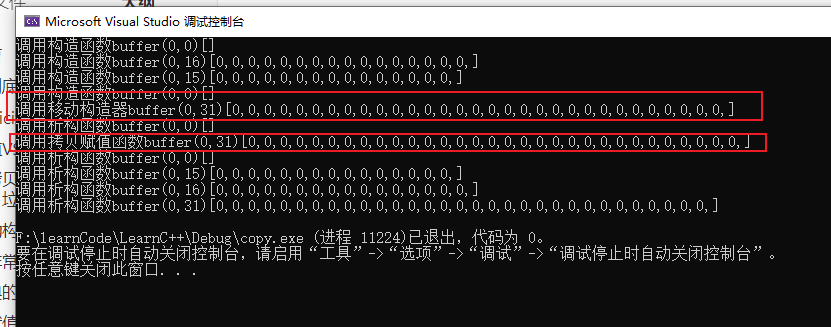### 运行结果

    auto buffers = std::vector<buffer>();
buffers.push_back(buffer(10));
buffers.push_back(buffer(9));
buffers.push_back(buffer(8));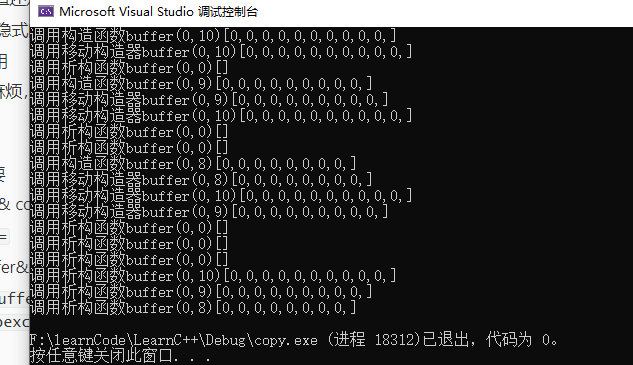C++ 天下第一